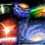# A request to the Brilliant staff. Problems in editing solutions.

Like a option to post images while solving problems there should also be an option to post images in solutions . There is a problem while editing solutions , if any small minor changes are to be done in solutions then the whole solution has to be written again because when we click edit , the format of solution changes and lots of extra "\" are added to unwanted places. e.g

this is a solution i posted and want to edit it .

let at some time t the velocity of the center of mass of the rolling part be v and radius be r. let there be p turns in the carpet per unit length.Let the current be then be I Let the total number of turns be n

\\\phi=B\.A=\\int B\\pi r^\{2\}dN=\\int\_\{0\}^\{r\}B\\pi r^\{2\}pdr=\\frac\{\\pi Bpr^\{3\}\}\{3\} \\

\$\\frac\{d\\phi\}\{dt\}=\\pi pBr^\{2\}\\frac\{dr\}\{dt\}=mI \$/extract_itex] We know that the mass is constant let the mass per unit length of carpet be \$\\rho\\$ \$\\rho x\+\\rho\\int\_\{0\}^\{r\}2\\pi rpdr=k \\$ \$v=\\frac\{dx\}\{dt\} \\$ \$\-\\frac\{v\}\{2\\pi rp\}=\\frac\{dr\}\{dt\} \\$ \$i=\-\\frac\{Bvr\}\{2m\} \\$ finally all the carpet is rolled out and velocity finally is 0 after t=Ts applying energy conservation. MgR=\int_{0}^{t}I^{2}mdt=\int_{0}^{t}$$\\frac\{\-B^\{2\}v^\{2\}r^\{2\}\}\{2m\}^{2}mdt$ \$$\\frac\{\\int\_\{0\}^\{t\}v^\{2\}r^\{2\}dt\}\{4\\int\_\{0\}^\{t\}dt\}$=$\\frac\{MmgR\}\{B^\{2\}T\}$=29$Note by Milun Moghe 7 years, 5 months ago This discussion board is a place to discuss our Daily Challenges and the math and science related to those challenges. Explanations are more than just a solution — they should explain the steps and thinking strategies that you used to obtain the solution. Comments should further the discussion of math and science. When posting on Brilliant: • Use the emojis to react to an explanation, whether you're congratulating a job well done , or just really confused . • Ask specific questions about the challenge or the steps in somebody's explanation. Well-posed questions can add a lot to the discussion, but posting "I don't understand!" doesn't help anyone. • Try to contribute something new to the discussion, whether it is an extension, generalization or other idea related to the challenge. • Stay on topic — we're all here to learn more about math and science, not to hear about your favorite get-rich-quick scheme or current world events. MarkdownAppears as *italics* or _italics_ italics **bold** or __bold__ bold - bulleted- list • bulleted • list 1. numbered2. list 1. numbered 2. list Note: you must add a full line of space before and after lists for them to show up correctly paragraph 1paragraph 2 paragraph 1 paragraph 2 [example link](https://brilliant.org)example link > This is a quote This is a quote  # I indented these lines # 4 spaces, and now they show # up as a code block. print "hello world" # I indented these lines # 4 spaces, and now they show # up as a code block. print "hello world" MathAppears as Remember to wrap math in \( ... $$ or $ ... $ to ensure proper formatting.
2 \times 3 $2 \times 3$
2^{34} $2^{34}$
a_{i-1} $a_{i-1}$
\frac{2}{3} $\frac{2}{3}$
\sqrt{2} $\sqrt{2}$
\sum_{i=1}^3 $\sum_{i=1}^3$
\sin \theta $\sin \theta$
\boxed{123} $\boxed{123}$

Sort by:

We are aware of this and working to fix the bug.

Staff - 7 years, 5 months ago

thank you for looking into this matter

- 7 years, 5 months ago

With regards to adding images, you can do so using markdown. Upload your image to your favorite image hosting site, like Imgur or Flickr, and then use the following code, without spaces:

Staff - 7 years, 4 months ago

Thank you for the support , ill try it out

- 7 years, 4 months ago